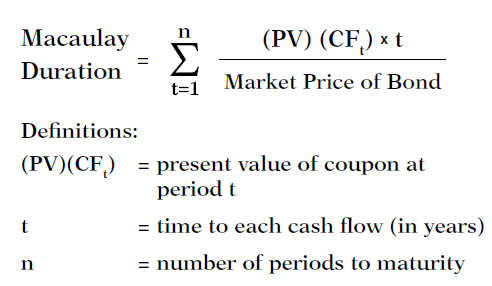# YTM Assistance

Studying yield to maturity on bonds. If the amount of the bond is 125M euro, the issue price = 99.731%, maturity = 7 years and the annual coupon is 5.5% on annual installments, how would I calculate the yield of maturity, the modified duration and duration?

YTM on the BAII

2nd CLR TVM
P/Y=C/Y=1
N 7 PV -99.731 PMT 5.5 FV 100 CPT I 5.547415009%

Thanks breadmaker, but I’m lost. I see 5.547 is the YTM but I don’t understand how you got there. How is the modified duration and duration calculated?

Annual interest at 5.547% equates the present value of all cash flows to the bond price of 99.731.